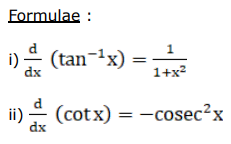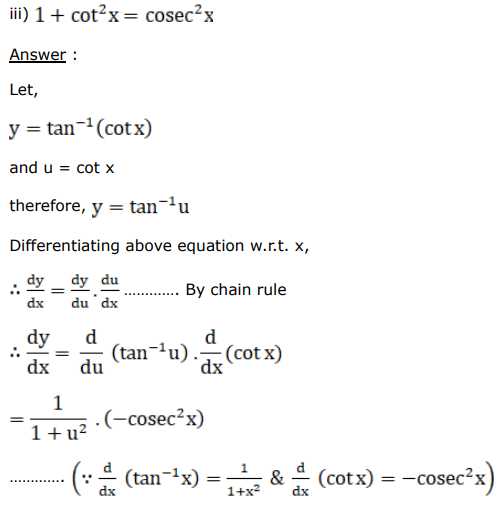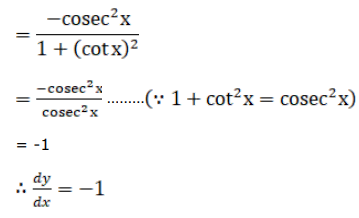# Differentiate each of the following w.r.t.Question:

Differentiate each of the following w.r.t. $x$ :

$\tan ^{-1}(\cot x)$

Solution: